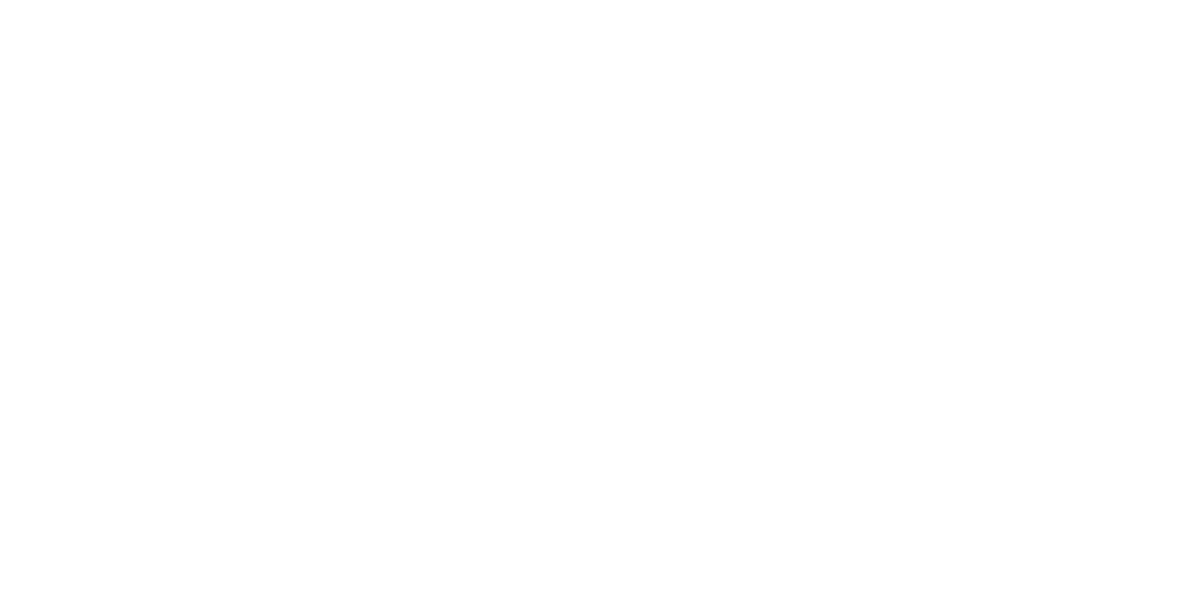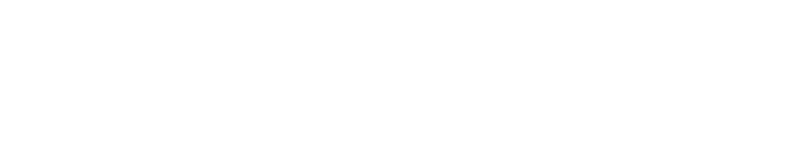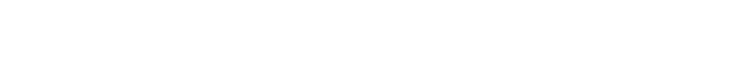Assessment-quiz
level IIIDo not use anything but your knowledge, pen and paper. This quiz has only one purpose: to give you a suggestion on which course to do.

Just in case, there might be several correct answers to the question. You need to select all of them to get the question right!
 Start the quiz
Which of the following can be counted as a correct solution to the following problem: What is the biggest possible number of kings can be placed on a chessboard in such a way that none of them attack each other?" Feel free to search for how the chess king moves if you do not know how.Next
 Submit
 Show the results
How many 7-digits numbers are there such that its digits are in strictly decreasing order? (e.g a number 9764310 is one of such numbers)
 Next
 Submit
 Show the results
There is a graph G on 100 vertices such that it is possible to delete one of its edges to get two connected components, each of which is a tree. How many edges can G have?
 Next
 Submit
 Show the results
Let A be the number of integers less than 1000000 such that the sum of their digits is 18, and let B the number of integers less than 1000000 such that the sum of their digits is 36. What is the value of (A - B)?
 Next
 Submit
 Show the results
Which of the following statements are correct? Don't forget to select all that apply!
Nope, S must be equal to 26
The parity of 1 + 4 + 9 + 16 + 25 + .... + 10000 is the same as the parity of 1 + 2 + 3 + 4 + ... + 100 = 5050 which is an even number
 Next
 Submit
 Show the results
How many zeros does the number 100! end with?
 Next
 Submit
 Show the results
Which of the statements on the picture are true?Next
 Submit
 Show the results
What is the value of the expression on the picture?Next
 Submit
 Show the results
Initially the number 7^2022 (=7 x 7 x 7 x 7 x ... x 7 (2022) times) is stored in the memory of a computer. Each minute the number in the memory is replaced by the sum of its digits. After 30 minutes, which number will the computer have in its memory?
 Next
 Submit
 Show the results
Among the statements below select those that are true:
 Next
 Submit
 Show the results
Please have a look at "Two in one: Maths for IT and discrete maths" course. It seems like the best fit for you. Click here.
Do not worry about the result, it is all fine. We just want to find you a suitable course. The courses #3A,#3B and #3C seem to be too hard for you, therefore we suggest you look at "Two in one: Maths for IT and discrete maths" (course #3). If you did not do the previous quiz well enough, then actually you might even need to do "Basic Olympiad Ideas" course. Check the content of the courses and make your final decision.
Please have a look at "Two in one: Maths for IT and discrete maths" course. It seems like the best fit for you. Click here.
Do not worry about the result, it is all fine. We just want to find you a suitable course. The courses #3A,#3B and #3C seem to be too hard for you, therefore we suggest you look at "Two in one: Maths for IT and discrete maths". This is the course#2.
Please have a look at courses #3A, #3B, #3C. We recommend you do courses #3A and #3C at least. As for #3B ("Diving in Geometry") -- take it if you have less than a year of experience with geometry.